# Progression

In this mini-lesson, we will explore the world of progression in math.

You will get to learn about progression meaning, progression in math, progression examples, types of progression, and other interesting facts around the topic.

You can also check out the playful calculators to know more about the lesson and try your hand at solving a few interesting practice questions at the end of the page.

Emma has a bracelet with 9 beads in two shapes.

Look at the bracelet shown below.Observe that the shapes - square and circle, follow a pattern.

Now, look at the sequence below.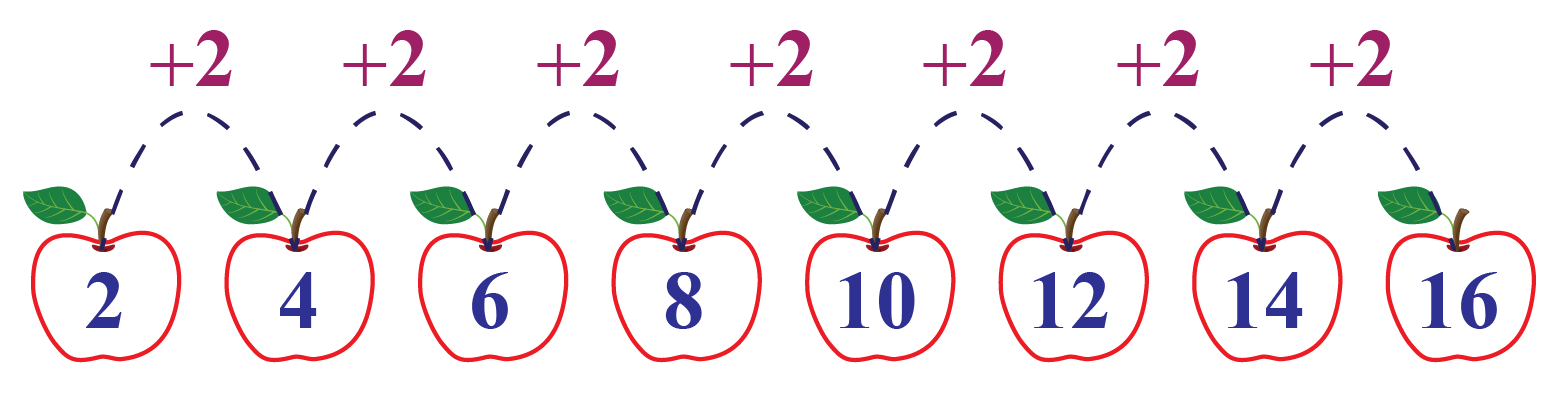These numbers are in ascending order.

Observe that each term is 2 more than the term preceding it.

In the sequence shown below, each term is 4 less than the term preceding it.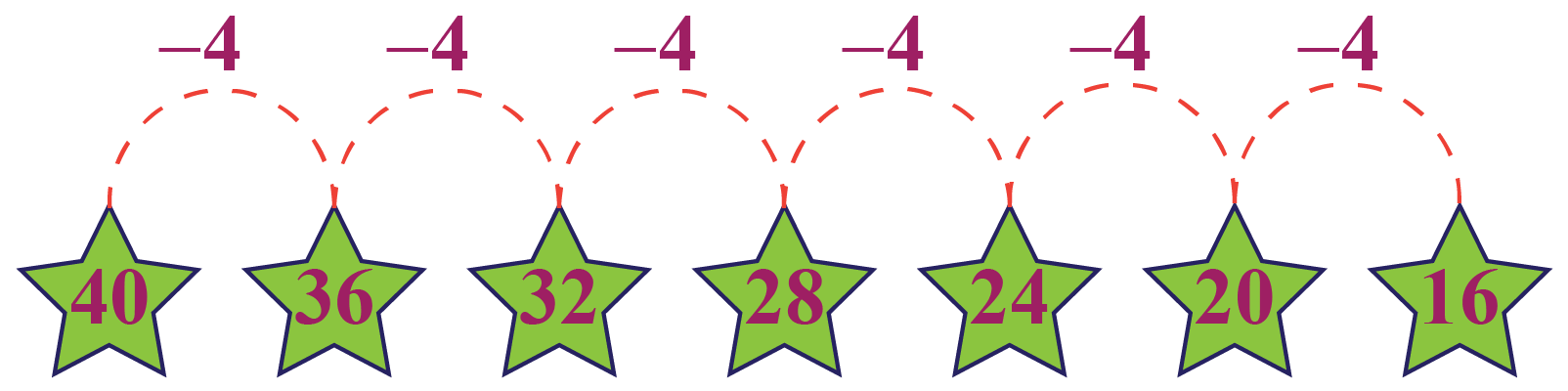All these form a pattern that shows a progression exhibited by the numbers.

## Lesson Plan

 1 What Is the Meaning of Progression? 2 Important Notes on Progression 3 Solved Examples on Progression 4 Thinking Out of the Box! 5 Interactive Questions on Progression

## What Is the Meaning of Progression?

Progression is a list of numbers (or items) that exhibit a particular pattern.

The difference between a sequence and a progression is that to calculate its $$n^{th}$$ term, a progression has a specific formula.

### Examples

Olivia has been offered a job with a starting monthly salary of $1000 with an annual increment of$200.Can you calculate her monthly salary for the next few years?

It will be $$\1000,\;\1200,\;\1400,\;\1600,\;\1800,\;\2000...$$

The $$n^{th}$$ term of the above sequence can be calculated by the formula $$\1000+\200(n-1)$$.

Here $$n$$ represents the number of years for which her salary is to be calculated.

Therefore, Olivia's salary over a number of years forms a progression.

## What Are the Types of Progression?

The three types of progression in maths are shown below.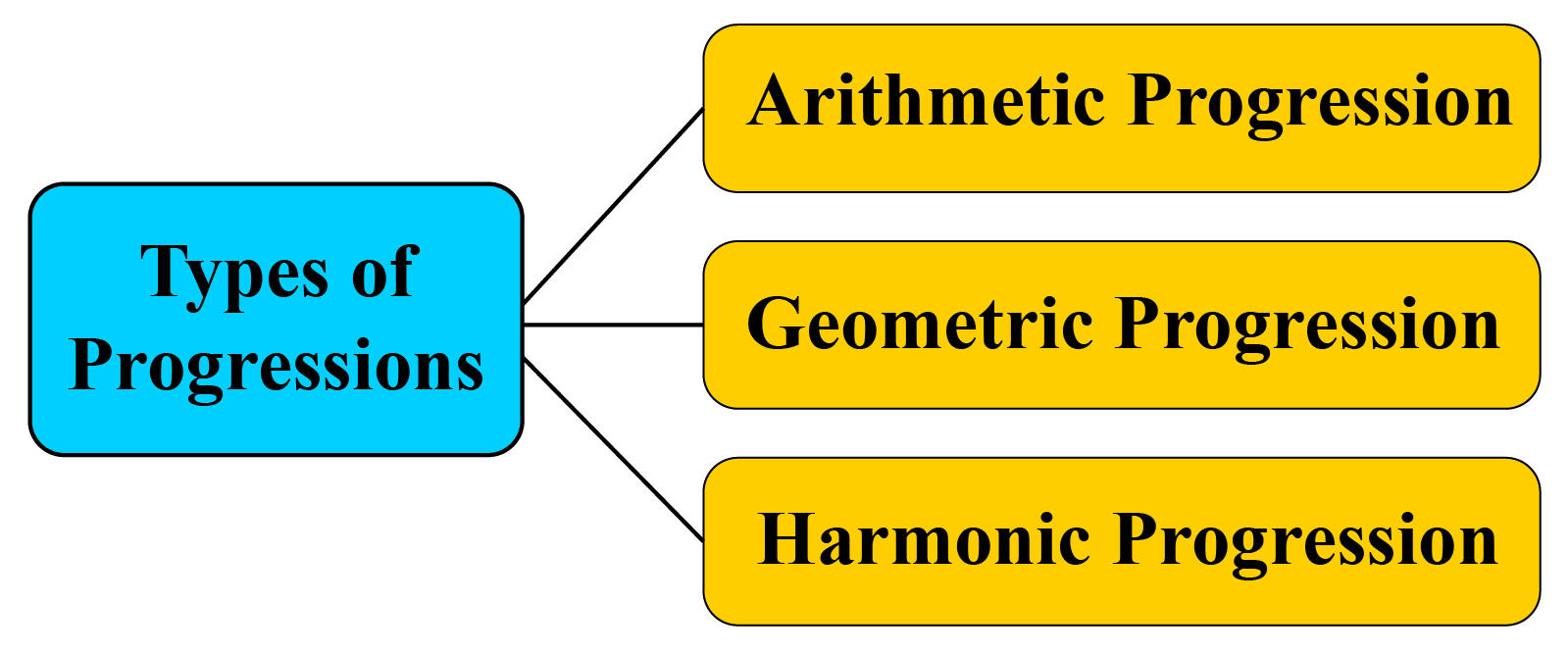Types of Progression Examples
Arithmetic Progression 1, 4, 7, 10, ...
Geometric Progression 4, 16, 64, 256, ...
Harmonic Progression $$\dfrac{1}{2}$$, $$\dfrac{1}{4}$$, $$\dfrac{1}{6}$$, ...

## Types of Progression

### Arithmetic Progression

An arithmetic progression is a sequence of numbers in which each successive term is the sum of its preceding term and a fixed number.

This fixed number is called a common difference.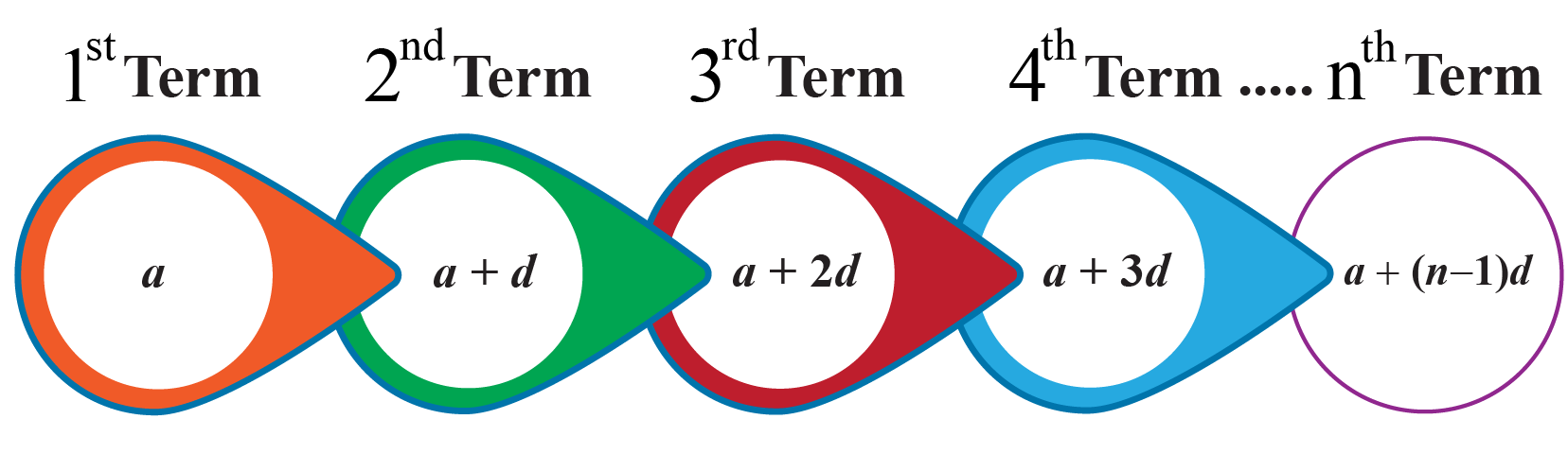### Arithmetic Progression Formulas

Let the first term of the progression be $$a$$, the common difference be $$d$$, and the $$n^{\text{th}}$$ term be $$a_n$$.

Then, the arithmetic progression formulas are given by:

 \begin{align}d&=a_{n}-a_{n-1}\\a_{n}&=a+(n-1)d\end{align}

### Arithmetic Progression Examples

For example, Minnie put $30 in her piggy bank when she was 7 years old. She increased the amount she put in her piggy bank on each successive birthday by$3.So, the amount in her piggy bank follows the pattern of $30,$33, $36, and so on. The succeeding terms are obtained by adding a fixed number, that is,$3.

This fixed number is called the common difference.

It can be positive, negative, or zero.

### Geometric Progression

A geometric progression is a sequence where every term bears a constant ratio to its preceding term.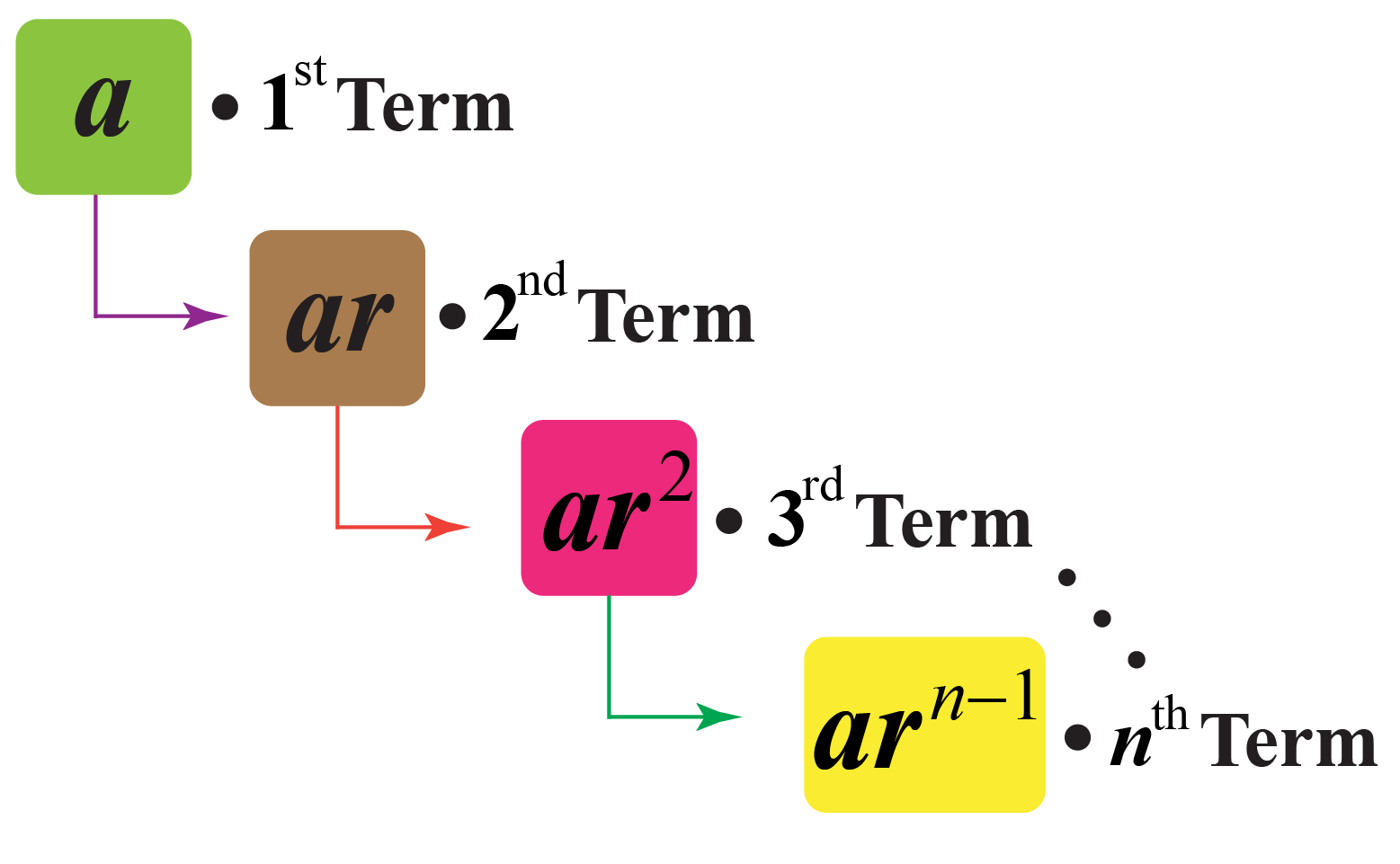### Geometric Progression Formulas

Let the first term of the progression be $$a$$, the common ratio be $$r$$, and the $$n^{\text{th}}$$ term be $$a_n$$.

Then, the geometric progression formulas are given by:

 \begin{align}r&=\dfrac{a_{n}}{a_{n-1}}\\a_{n}&=ar^{n-1}\end{align}

### Geometric Progression Examples

Consider an example of a geometric sequence: 1, 4, 16, 64, ...

Observe that $$\dfrac{4}{1}=\dfrac{16}{4}=\dfrac{64}{16}=4$$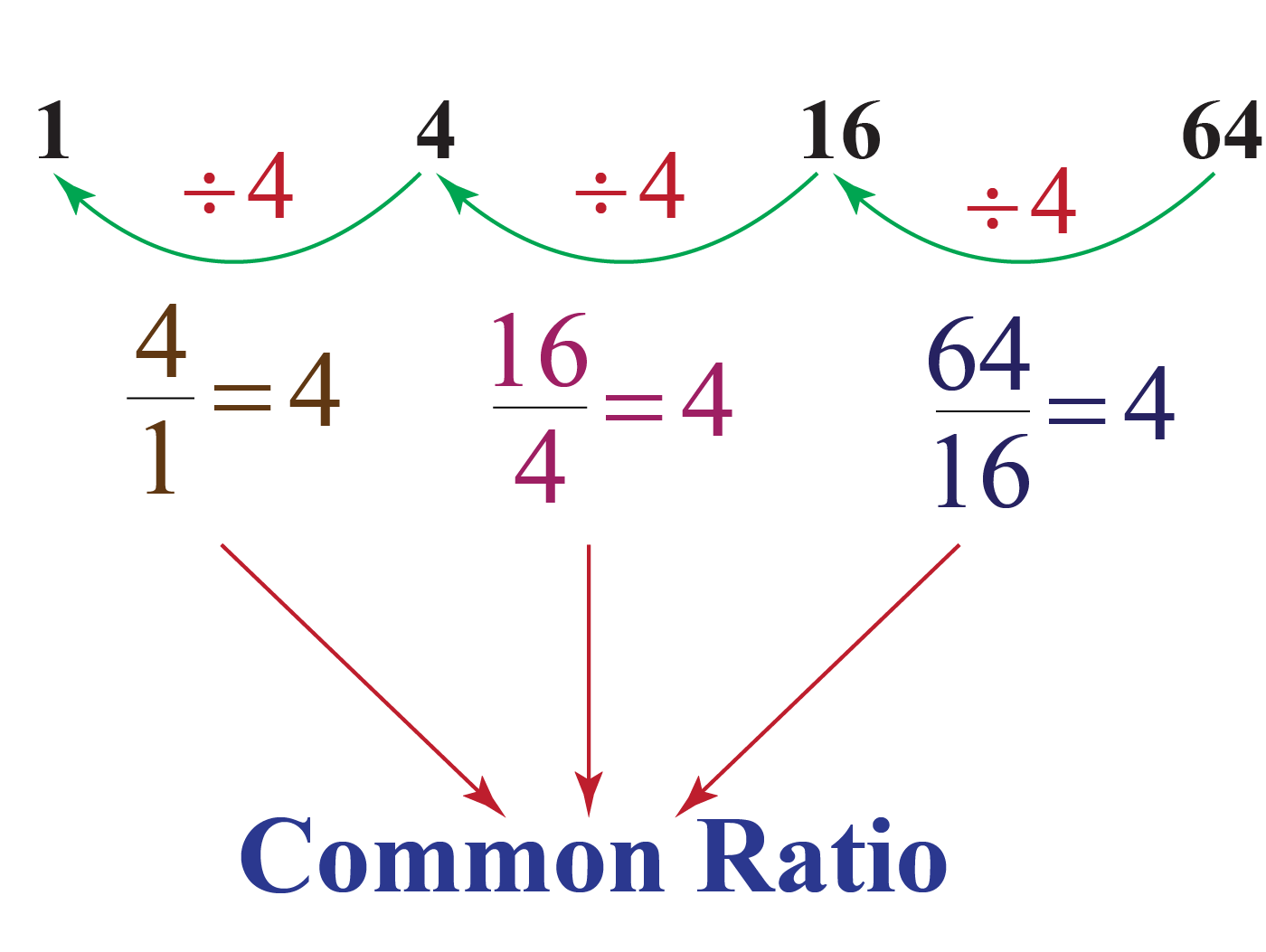Here, 4 is the common ratio.

### Harmonic Progression

A harmonic progression is a sequence obtained by taking the reciprocal of the terms of an arithmetic progression.

The sequence of natural numbers is an arithmetic progression.

So, taking reciprocals of each term we get:

$1, \dfrac{1}{2}, \dfrac{1}{3}, \dfrac{1}{4}, ...$

This is harmonic progression.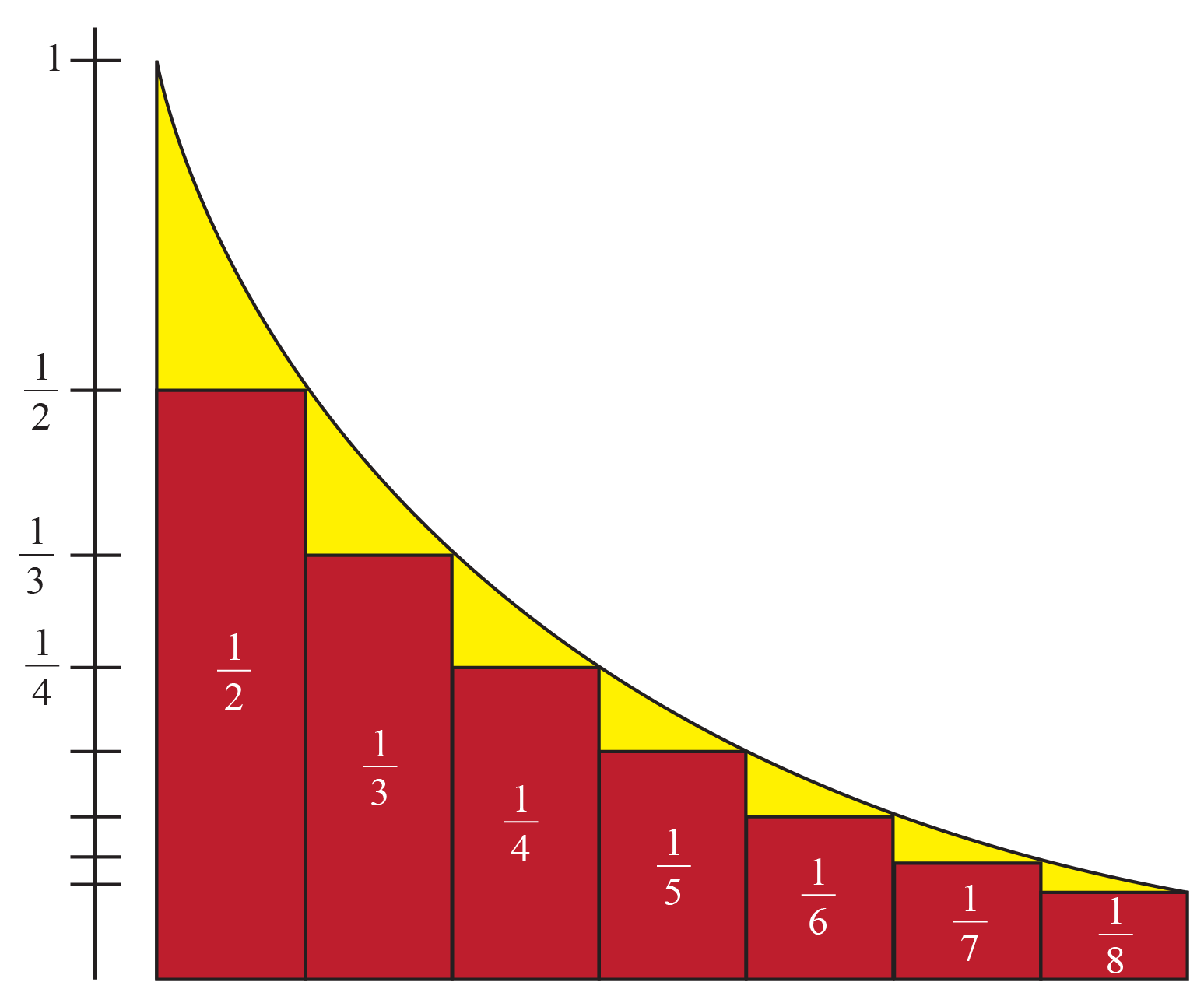### Harmonic Progression Formulas

Let the first term of the sequence be $$a$$, the common ratio be $$r$$, and the $$n^{\text{th}}$$ term be $$a_n$$.

Then, the harmonic progression formulas are given by:

 \begin{align}d&=a_{n-1}-a_{n}\\a_{n}&=\dfrac{1}{a+(n-1)d}\end{align}

### Harmonic Progression Examples

When a ball is dropped, the initial height reached by the ball is $$\dfrac{1}{2}$$ units and after the first impact, the height attained by the ball is $$\dfrac{1}{4}$$ units.

After the second impact, the height attained by the ball is $$\dfrac{1}{6}$$ units.

After the third impact, the height attained by the ball is $$\dfrac{1}{8}$$ units, and so on.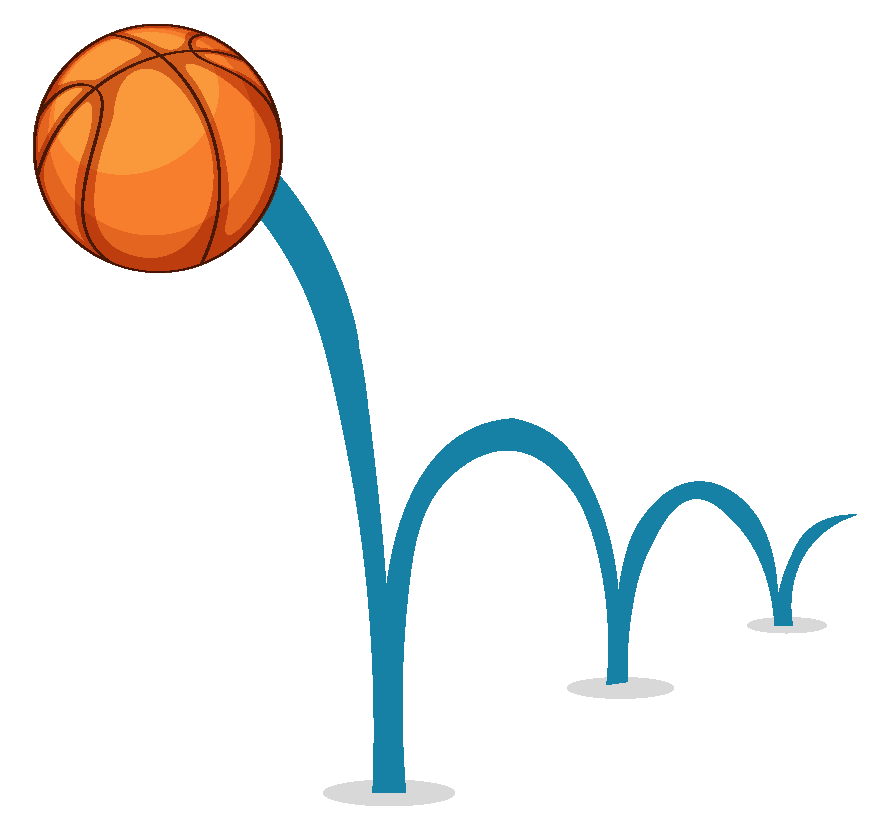Now the sequence of heights formed by the ball is: $$\dfrac{1}{2},\;\dfrac{1}{4},\;\dfrac{1}{6},\;\dfrac{1}{8},...$$

This sequence is a harmonic progression because the reciprocal of all the terms of this progression form an arithmetic progression.

Reciprocal of the sequence: $$2,\;4,\;6,\;8,...\to A.P.$$

Hence, the sequence $$\dfrac{1}{2},\;\dfrac{1}{4},\;\dfrac{1}{6},\;\dfrac{1}{8},...\to H.P.$$

## Solved Examples

 Example 1

A taxi charges $2 for the first mile and$1.5 for each subsequent mile.How much will Katie need to pay the taxi driver if she travels 5 miles?

Solution

The taxi fare for the first few miles are $2,$3.5, \$5, ...

It forms an arithmetic sequence with a common difference, $$d=1.5$$, and the first term, $$a=2$$

The 5th term of the sequence will represent the taxi fare for the first 5 miles.

The 5th term of the sequence will be given by $$a+4d$$.

\begin{align}a+4d&=2+4(1.5)\\&=2+6\\&=8\end{align}

 $$\therefore$$ Katie pays $$8$$ to the taxi driver for 5 miles.
 Example 2

Hailey's teacher asks her to find the $$10^{\text{th}}$$ term of the sequence: 1, 3, 9, 27, ...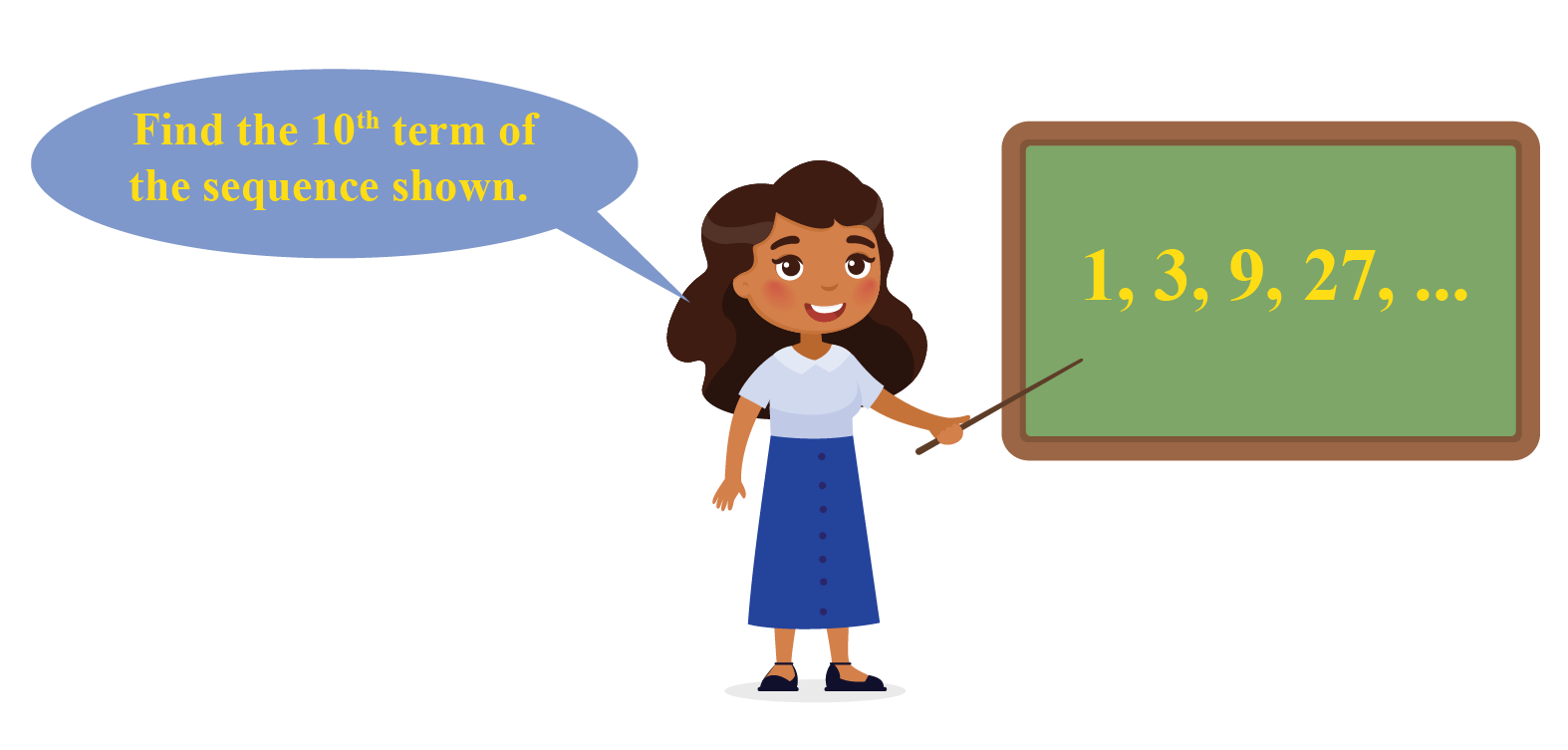Can you help her?

Solution

Observe that $$\dfrac{3}{1}=\dfrac{9}{3}=\dfrac{27}{9}=3$$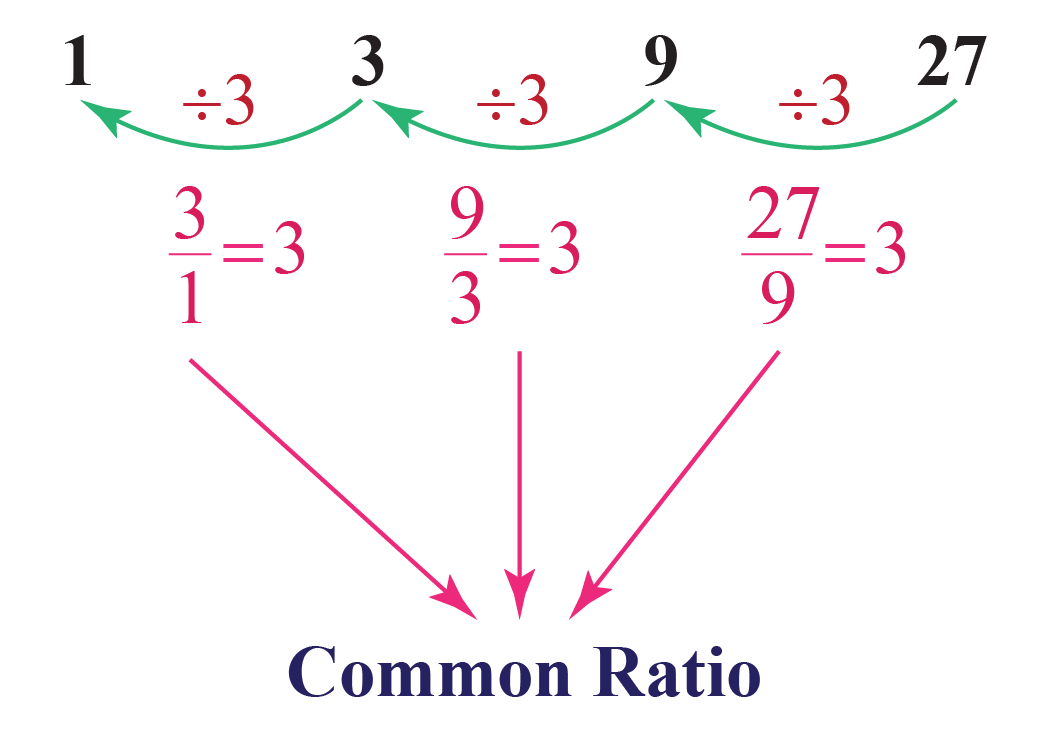Here, $$r=3$$ is a common ratio.

Hence, the given sequence represents a geometric progression.

The 10th term of the sequence will be given by $$ar^{9}$$.

\begin{align}ar^{9}&=1\times 3^9\\&=3^9\end{align}

 $$\therefore$$ The 10th term of the sequence is $$3^9$$.
 Example 3

Look at the pattern shown below.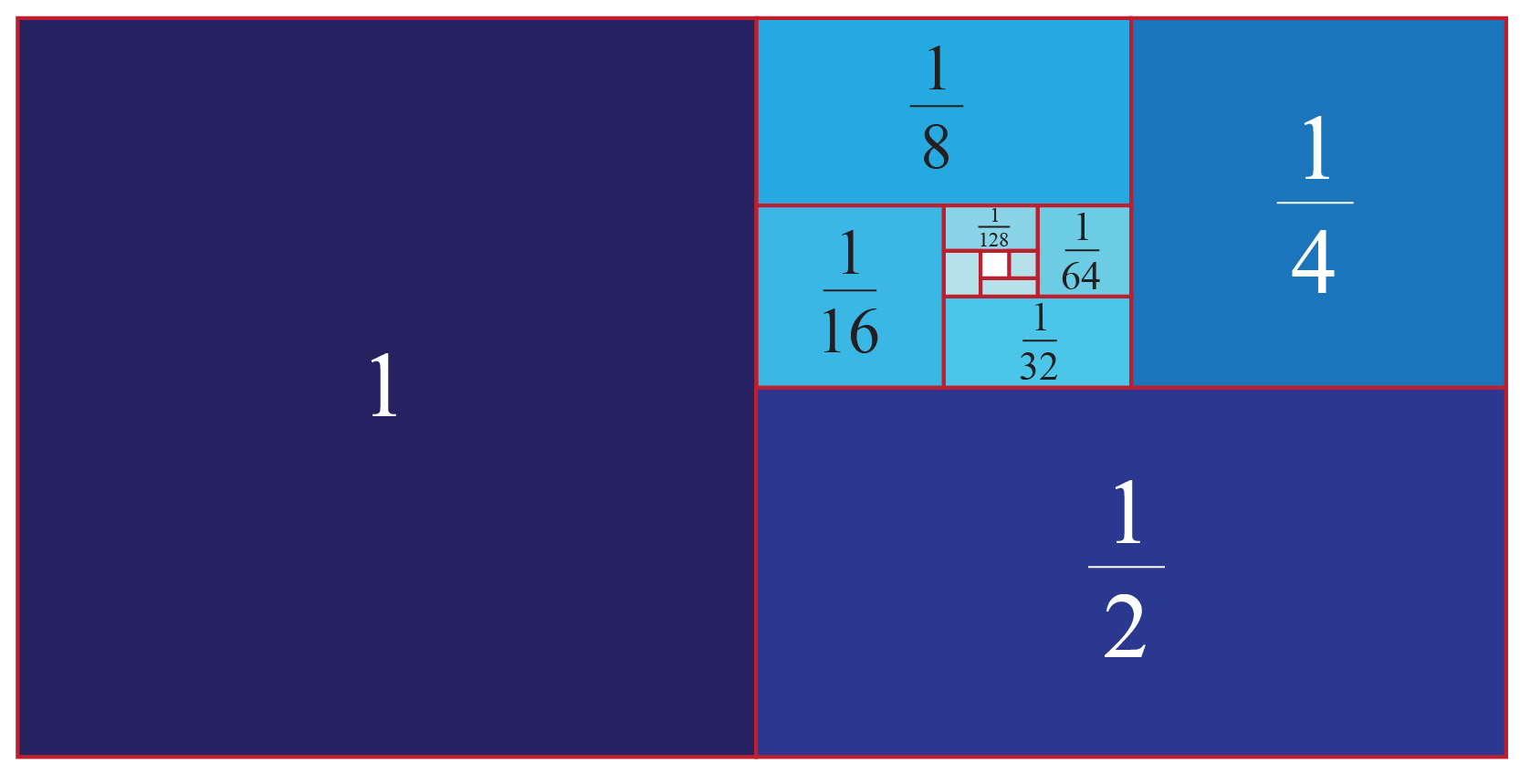Observe that each box is half the size of the box next to it.

Which sequence does this pattern represent?

### Solution

Let's write the sequence represented in the figure.

$1, \dfrac{1}{2}, \dfrac{1}{4}, \dfrac{1}{8}, \dfrac{1}{16},...$

Every successive term is obtained by dividing its preceding term by 2

The sequence exhibits a common ratio of $$\dfrac{1}{2}$$

 $$\therefore$$ The pattern represents a geometric sequence.
 Example 4

Which term of the A.P. $$3, 8, 13, 18,...$$ is $$73$$?

Solution

The given sequence is:

$3,8,13,18,...$

Here, the first term is,

$a=3$

The common difference is,

$d = 8-3= 13-8=...=5$

Let us assume that the $$n^{th}$$ term is,

$a_n=73$

Substitute all these values in the general term of an AP:

\begin{align} a_n &= a+(n-1)d \\[0.3cm] 73 &= 3+(n-1)5\\[0.3cm] 73&=3+5n-5\\[0.3cm] 73&= 5n -2 \\[0.3cm] 75 &=5n\\[0.3cm] 15&=n \end{align}

 $$\therefore 73 \text{ is the } 15^{th} \text{ term}$$
 Example 5

The 6th term and the 11th term of a harmonic sequence are 10 and 18 respectively.

Can you find the common difference between the associated arithmetic sequence?

Solution

By definition of the harmonic sequence, we have

6th term of arithmetic sequence = $$\dfrac{1}{10}$$

11th term of arithmetic sequence = $$\dfrac{1}{18}$$

By definition of an arithmetic sequence, we have 2 equations.

\begin{align}a+5d=\dfrac{1}{10}\;\;\;\;\;\; \cdots (1)\end{align}

\begin{align}a+10d=\dfrac{1}{18}\;\;\;\;\;\; \cdots (2)\end{align}

Subtract equation (1) from equation (2)

\begin{align}(a+10d)-(a+5d)&=\dfrac{1}{18}-\dfrac{1}{10}\\5d&=-\dfrac{2}{45}\\d&=-\dfrac{2}{225}\end{align}

 $$\therefore$$ The common difference is $$-\dfrac{2}{225}$$Think Tank
 1. There are 25 trees at equal distances of 5 feet in a line along with a well. The distance of the well from the nearest tree is 10 feet. A gardener waters all the trees separately starting from the well and he returns to the well after watering each tree. Find the total distance the gardener will cover.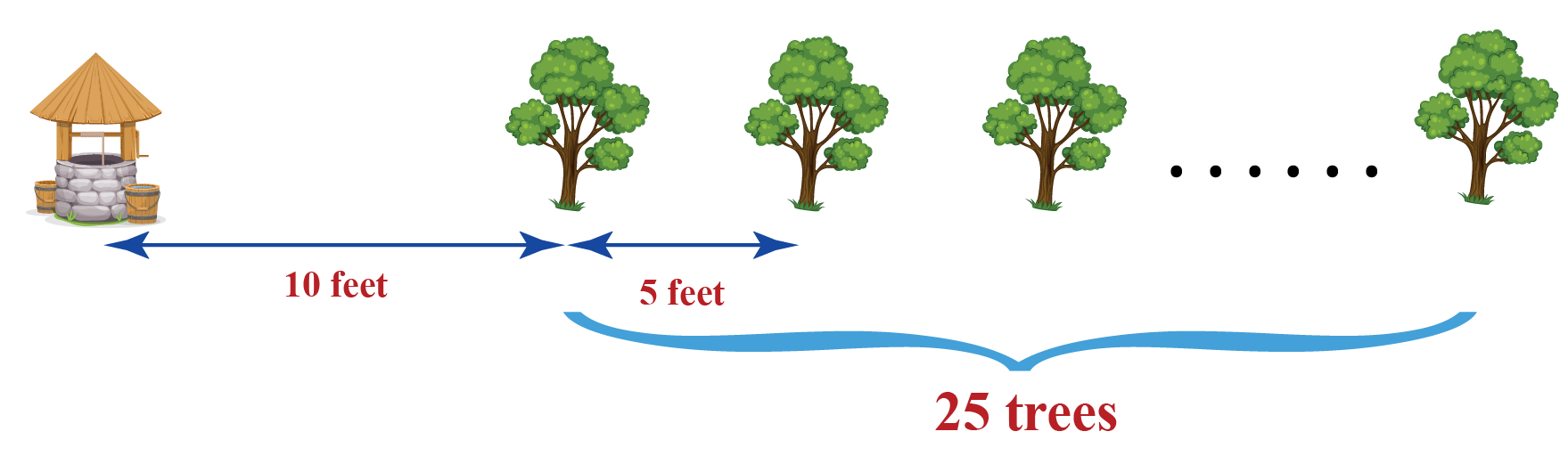## Interactive Questions

Here are a few activities for you to practice.

## Let's Summarize

We hope you enjoyed learning about progression with the examples and practice questions. Now, you will be able to easily remember progression meaning, progression in math, progression examples, types of progression, which include arithmetic progression, geometric progression, and harmonic progression.

At Cuemath, our team of math experts is dedicated to making learning fun for our favorite readers, the students!

Through an interactive and engaging learning-teaching-learning approach, the teachers explore all angles of a topic.

Be it worksheets, online classes, doubt sessions, or any other form of relation, it’s the logical thinking and smart learning approach that we, at Cuemath, believe in.

### 1. What is progression?

Progression is a list of numbers (or items) that exhibit a particular pattern and also has a particular formula for its $$n^{th}$$ term.

### 2. What is the difference between progression and series?

The difference between a series and a progression is that to calculate its $$n^{th}$$ term, a progression has a specific formula.

### 3. Is a progression a sequence?

Yes, a progression is always a sequence.

### 4. What is the difference between sequence and progression?

The difference between a sequence and a progression is that to calculate its $$n^{th}$$ term, a progression has a specific formula.

### 5. How many types of progressions are there?

There are three types of progressions.

1. Arithmetic progression
2. Geometric progression
3. Harmonic progression

### 6. How do you calculate progression?

Progressions are calculated either by using arithmetic progression formulas, geometric progression formulas, or by using harmonic progression formulas.

### 7. What is arithmetic progression in simple words?

An arithmetic progression is a sequence of numbers in which each successive term is the sum of its preceding term and a fixed number.

This fixed number is called a common difference.

### 8. What is the formula of geometric progression?

The formula for the $$n^{th}$$ term of a geometric progression is $a_{n}=ar^{n-1}$

### 9. What is the meaning of HP in math?

In mathematics, HP stands for harmonic progression.

### 10. Can a sequence be both geometric and arithmetic?

Yes, a sequence can be both geometric and arithmetic.

For example: a constant sequence $$1,1,1,1,...$$ is both geometric and arithmetic.

More Important Topics
Numbers
Algebra
Geometry
Measurement
Money
Data
Trigonometry
Calculus